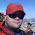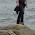## Thursday, June 3, 2010

### Stuff that Shouldn't Work

Making practice or test exercises is harder than you might first think before becoming a teacher. If you ever make a problem up on the fly while lecturing, it's highly probable that you'll create something with hideous fractions, irrational or imaginary numbers, extraneous solutions, etc., that you didn't want, which winds up sidetracking you from the point you were trying to make.

Another pitfall is creating problems that are singularities, i.e., the correct answer can be produced by some completely incorrect process, one that won't work for any other problem of the same nature. For remedial math students, this is almost a nightmare scenario, since their capacity to correctly generalize from the specific to the abstract is already shaky and confused as it is.

As just one example, here's one of my favorites from the algebra workbook we use at my school (custom edition produced by other teachers in same department):
If 1.05x = 22.05, then x = ?
Now, the correct process is to divide both sides by 1.05, and see that x = 22.05/1.05 = 21. But horribly, if a student mistakenly subtracts 1.05, then they also get the same answer! Say x = 22.05 - 1.05 = 21. Thus, this exercise allows a student to "submarine" a totally broken process (answers are multiple-choice in the book), giving them apparent confirmation that they're doing the right thing when they're absolutely not. (Note that this particular exercise was changed in a newer edition after I pointed it out.)

Enough prelude. The thing I'm trying to get around to is that last night I saw the "crown jewel" for this kind of problem, as part of a set of practice problems for the ACT Compass Test in Algebra. (You can actually see it here: "Sample Math Test Questions: Numerical Skills/Pre-Algebra and Algebra", Algebra item #14). Something like this:
For x ≠ 3, reduce (x2 - 9)/(x-3).
Now, the point of this exercise is to practice factoring (in this case, the top is a "difference of squares") and then cancel like factors on the top & bottom. Write: (x2 - 9)/(x-3) = (x+3)(x-3)/(x-3) = x+3.

But last night my students got all weirded out when I was writing that much (there's an additional wrinkle in the Compass problem, but it's not germane to my point) and said they got the right answer with a lot less work. They explained: "Divide x2 on top by x on bottom and get x. Divide -9 on top by -3 on bottom and get +3. There's the answer, x+3."

Now obviously this is a horribly mutilated process (and not uncommon!), thinking that you can divide individual terms in a rational expression piecemeal. (My best explanation, not that it gets fantastic traction, is always "Division distributes across addition, so if you divide by x, you have to divide every term by x." ) But the really crazy unique thing about this problem is that the broken process actually works for every possible problem of this format!

Consider all possible ways of constructing a "difference of squares" on top, and one of its canceling factors on the bottom. Case 1: Say you're reducing (a2-b2)/(a+b). Correct process: (a2-b2)/(a+b) = (a+b)(a-b)/(a+b) = a-b. Incorrect process: (a2-b2)/(a+b) = a2/a - b2/b = a-b (same answer). Case 2: Say you're reducing (a2-b2)/(a-b). Correct process: (a2-b2)/(a-b) = (a+b)(a-b)/(a-b) = a+b. Incorrect process: (a2-b2)/(a-b) = a2/a - b2/(-b) = a+b (also the same answer).

So not only does the "broken" process work for all permutations of this kind of problem, it even manages to get all the signs correct regardless of how those have been set up. Arrrghh!!!

(Silver lining: At least two of my students had the courage to tell me that's what they'd done, and I had the presence of mind to listen to it last night. I've used this practice test for about 5 years without anyone pointing out how they were doing it like that.)

1.I just finished a summer 2-week class on teaching number sense and we all agreed that detecting these "things that shouldn't work" issues requires absolute ninja number sense on the part of the teacher. My favorite was when the problem (either from the text or my own misguided brain) would lead students to 2 twos and a four, leaving students to guess if the twos were added, multiplied, or if two was squared.

2.Useful, thank you Daniel for pointing me here (from [https://matheducators.stackexchange.com/q/12368/3502])

3.Thank you Daniel for pointing me here. My problem arose under like circumstances as the ones leading you to write this blog entry. My test was multiple choice as well, thus students had no chance to document which prcedure the followed to the result. This is often a caveat in MC tests, as you often need to assess students' ability to process a problem - rather than their ability to conjure a right answer.

1.Thanks for checking my blog and your kind words! I totally agree; MC testing presents all kinds quagmires to understanding like this. (This is actually a common point of debate between colleagues in my department; some of whom want all-MC tests all the time.)